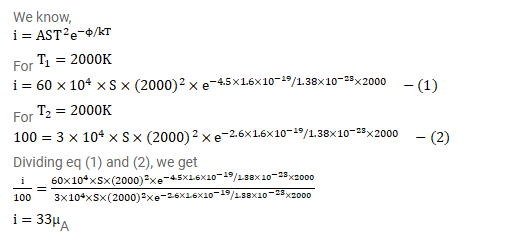# The saturation current from a thoriated-tungsten cathode at 2000K is 10mA.

Question:

The saturation current from a thoriated-tungsten cathode at $2000 \mathrm{~K}$ is $10 \mathrm{~mA}$. What will be the saturation current for puretungsten cathode of the same surface area operating at the same temperature? The constant A in the Richardson-Dushmaan

equation is $60 \times \frac{10^{4} \mathrm{~A}}{\mathrm{~m}^{2}}-\mathrm{K}^{2}$ for pure tungsten and $3.0 \times \frac{10^{4} \mathrm{~A}}{\mathrm{~m}^{2}}-\mathrm{K}^{2}$

for thoriated tungsten. The work function of pure tungsten is 4.5eV and that of thoriated tungsten is 2.6eV.

Solution: